# SAT II Math II : Probability

## Example Questions

### Example Question #1 : Probability

Two dice, one red and one blue, are altered. The red die comes up a "1"of the time and a "2"of the time, and the blue die comes up a "1"of the time and a "2"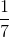of the time. What are the odds against rolling a "2", a "3", or a "4" with the two dice?

Insufficient information is given to solve the problem.

23 to 5

6 to 1

8 to 3

11 to 3

Insufficient information is given to solve the problem.

Explanation:

A "2" can only be rolled on the two dice with a double "1";, a "3", with a "1-2" or "2-1", and a "4", with a "1-3", a "2-2", or a "3-1". This makes six favoriable rolls. To answer the question, we must know the probabilities that the red die will come up "1", "2", and "3"; we must also know the same for the blue die. We know the probabilities for "1" and "2" for each die, but not "3". Therefore, insufficent information is given in the problem.

### Example Question #2 : Probability

What is the probability of choosing 2 67's in a row out of the following data set? Assume replacement.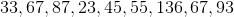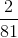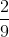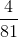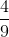Explanation:

What is the probability of choosing 2 67's in a row out of the following data set? Assume replacement.Let's begin by recalling that probability can be thought of as follows: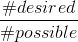Meaning that the probability of an event is the number of times the desired outcome exists, over the total number of outcomes.

In this case, each pick has two possible desired outcomes, and 9 total outcomes. (there are two 67's, and 9 total terms).

So the probability of each choice being a 67 is:However, we are not done yet. We need the probability of choosing two 67's in a row. Assuming replacement means that each probability will be the same, so to find the probability of events, we will multiply each probability together. This gets: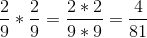### Example Question #3 : Probability

A fair coin is tossed 4 times. What is the total number ways the results can be observed (ie HHHH, HHHT, HHTH.....).Explanation:

In general, the number of outcomes that can be observed when flipping a coin is found using the formula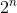where n is the number of tosses. In this case there are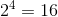ways to observe the outcome.

Alternatively, if n is small enough you can list out the possible outcomes:

1. HHHH

2. HHHT

3. HHTH

4. HTHH

5. THHH

6. HHTT

7. HTHT

8. HTTH

9. THTH

10. TTHH

11. THHT

12. TTTH

13. TTHT

14. THTT

15. HTTT

16. TTTT

### Example Question #4 : Probability

In a carnival game, a player must spin two independent spinners. If the first spinner has a probability oflanding on red, and the second spinner has a probability of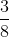landing on red, what is the probability of the first spinner NOT landing on red, and the second spinner landing on red?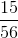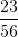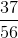Explanation:

Since the spinners are independent of each other, we will need to multiply the probability of not landing on red on the first spinner and the probability of landing on red on the second spinner together.

The probability of not landing on red for the first spinner is found by subtracting the probability of landing on red from.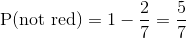Thus,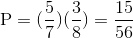### Example Question #5 : Probability

Suppose there is a bag of marbles.  There are two reds, two greens, and one orange marbles.  Without replacement, what is the probability of choosing a red, and another red?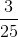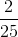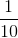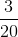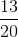Explanation:

There are five marbles altogether.

The first marble is a red, which is two of the five marbles in the bag.

The probability of choosing a red for the first trial is:Without replacement, there are four marbles remaining, and only one red marble remains.

The probability of choosing a red for the second trial is:Multiply the two probabilities.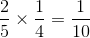The answer is: